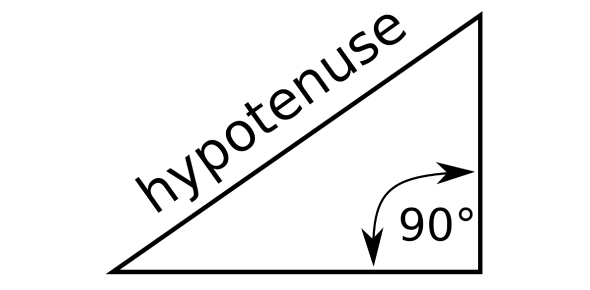What is the length of the hypotenuse of an isoceles right angled - ProProfs DiscussTopicsMoreProductsMore+ Ask Question

# What is the length of the hypotenuse of an isoceles right-angled triangle of side 12cm?

Asked by K. Tanaka, Last updated: Feb 14, 2020

###Request 0FollowShareAnswer AnonymouslyAnswer LaterCopy Link#### John F. connor

Traveler, Avid Reader, Free as a bird

John F. connor, Content Marketing executive, MA, Minsk,PolandAn isosceles triangle is a side where two of the sides are equal in length. These two sides are going to be the ones that meet at the right angle. The hypotenuse is the longer side of the triangle that is opposite of the right angle. Therefore, you already know that lengths of two sides of the triangle if one side is twelve centimeters. The Pythagorean Theorem has a formula of A squared plus B squared equal C squared.A and B are the equal sides on the isosceles triangle and C is the hypotenuse. So, twelve squared is equal to one hundred forty-four plus one-hundred forty-four which is the amount for B squared. The square root of two hundred eight-eight is sixteen point ninety-seven or almost 17 centimeters squared.Search for Google imagesSelect a recommended image
Upload from your computerCancelSearch for Google imagesSelect a recommended image
Upload from your computerCancelSearch for Google imagesSelect a recommended image
Upload from your computerCancel